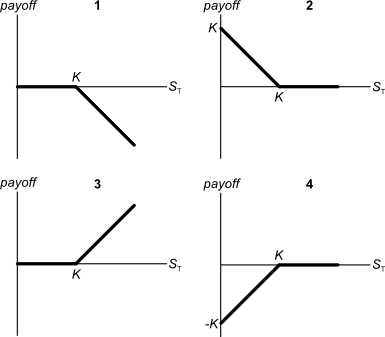# Fight Finance

#### CoursesTagsRandomAllRecentScores

The required return of a project is 10%, given as an effective annual rate.

What is the payback period of the project in years?

Assume that the cash flows shown in the table are received smoothly over the year. So the $121 at time 2 is actually earned smoothly from t=1 to t=2.  Project Cash Flows Time (yrs) Cash flow ($) 0 -100 1 11 2 121

A wholesale building supplies business offers credit to its customers. Customers are given 60 days to pay for their goods, but if they pay within 7 days they will get a 2% discount.

What is the effective interest rate implicit in the discount being offered?

Assume 365 days in a year and that all customers pay on either the 7th day or the 60th day. All rates given below are effective annual rates.

Below are 4 option graphs. Note that the y-axis is payoff at maturity (T). What options do they depict? List them in the order that they are numberedAn old company's Firm Free Cash Flow (FFCF, same as CFFA) is forecast in the graph below.To value the firm's assets, the terminal value needs to be calculated using the perpetuity with growth formula:

$$V_{\text{terminal, }t-1} = \dfrac{FFCF_{\text{terminal, }t}}{r-g}$$

Which point corresponds to the best time to calculate the terminal value?

Which of the below formulas gives the payoff $(f)$ at maturity $(T)$ from being long a put option? Let the underlying asset price at maturity be $S_T$ and the exercise price be $X_T$.

Mr Blue, Miss Red and Mrs Green are people with different utility functions. Which of the statements about the 3 utility functions is NOT correct?If a stock's future expected effective annual returns are log-normally distributed, what will be bigger, the stock's or effective annual return? Or would you expect them to be ?

You deposit money into a bank account. Which of the following statements about this deposit is NOT correct?

Question 852  gross domestic product growth, inflation, employment, no explanation

When the economy is booming (in an upswing), you tend to see:

Question 860  idiom, hedging, speculation, arbitrage, market making, insider trading, no explanation

Which class of derivatives market trader is NOT principally focused on ‘buying low and selling high’?